# Dynamic trees Steator and Tarjan 83 1 Operations

• Slides: 26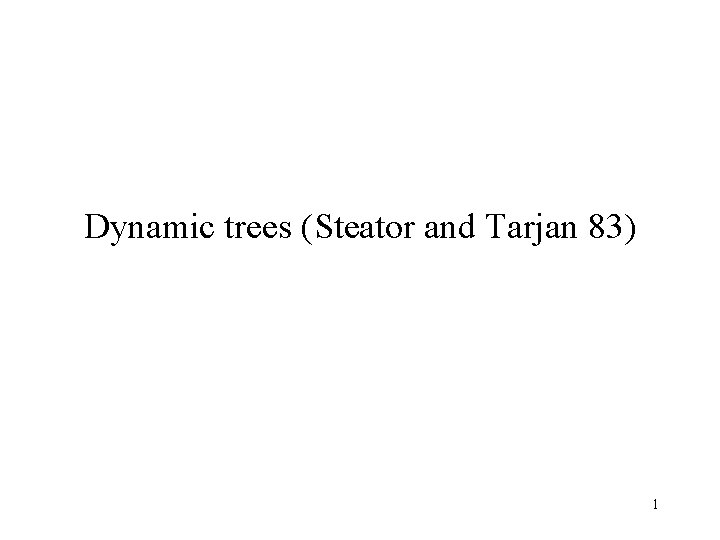Dynamic trees (Steator and Tarjan 83) 1Operations that we do on the trees Maketree(v) w = findroot(v) (v, c) = mincost(v) addcost(v, c) link(v, w, r(v, w)) cut(v) findcost(v) 2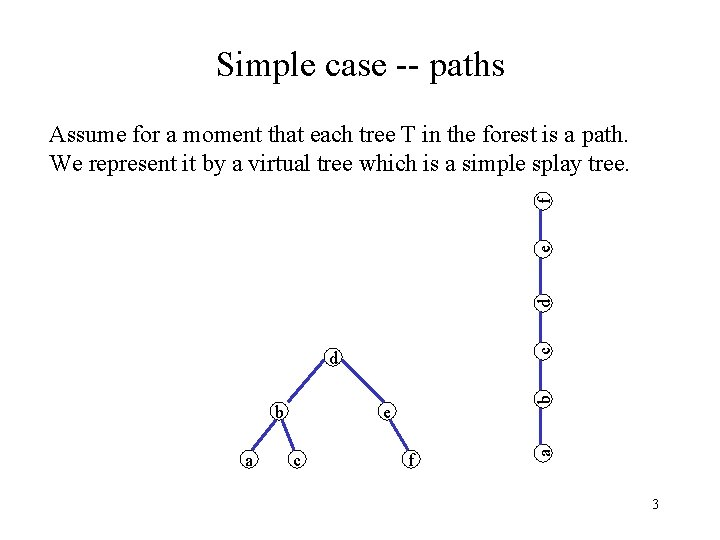Simple case -- paths c d e f Assume for a moment that each tree T in the forest is a path. We represent it by a virtual tree which is a simple splay tree. a e c f a b b d 3Findroot(v( Splay at v, then follow right pointers until you reach the last vertex w on the right path. Return w and splay at w. 4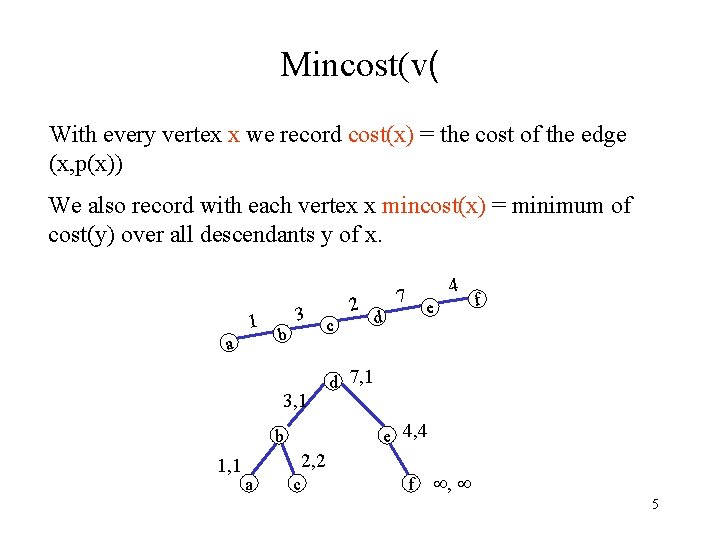Mincost(v( With every vertex x we record cost(x) = the cost of the edge (x, p(x)) We also record with each vertex x mincost(x) = minimum of cost(y) over all descendants y of x. 1 a b 3 3, 1 c e d d 7, 1 2, 2 a f e 4, 4 b 1, 1 2 4 7 c f , 5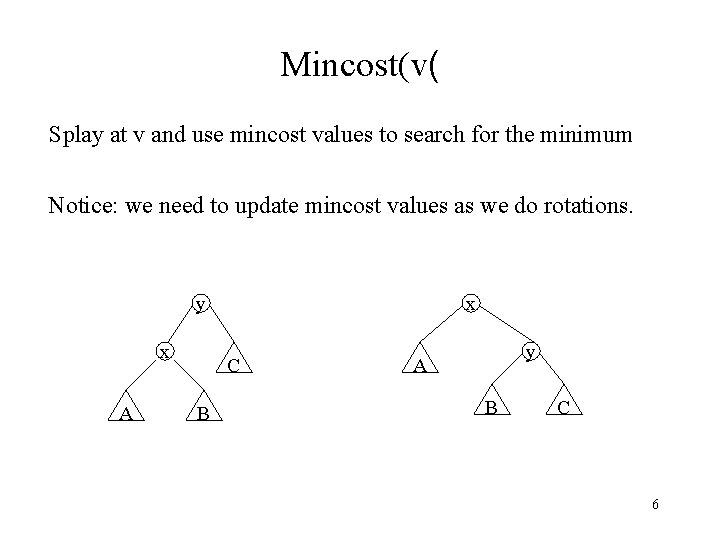Mincost(v( Splay at v and use mincost values to search for the minimum Notice: we need to update mincost values as we do rotations. y x A x C B y A B C 6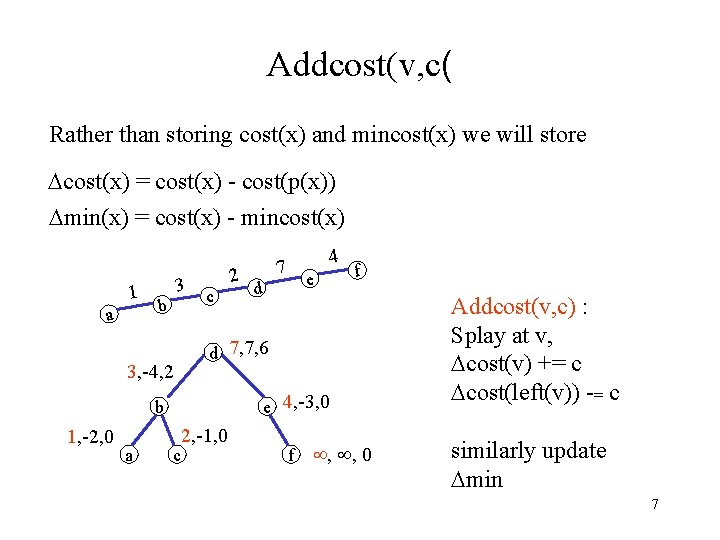Addcost(v, c( Rather than storing cost(x) and mincost(x) we will store cost(x) = cost(x) - cost(p(x)) min(x) = cost(x) - mincost(x) 1 a 3 b 3, -4, 2 2 c a e d f d 7, 7, 6 e 4, -3, 0 b 1, -2, 0 4 7 c 2, -1, 0 f , , 0 Addcost(v, c) : Splay at v, cost(v) += c cost(left(v)) -= c similarly update min 7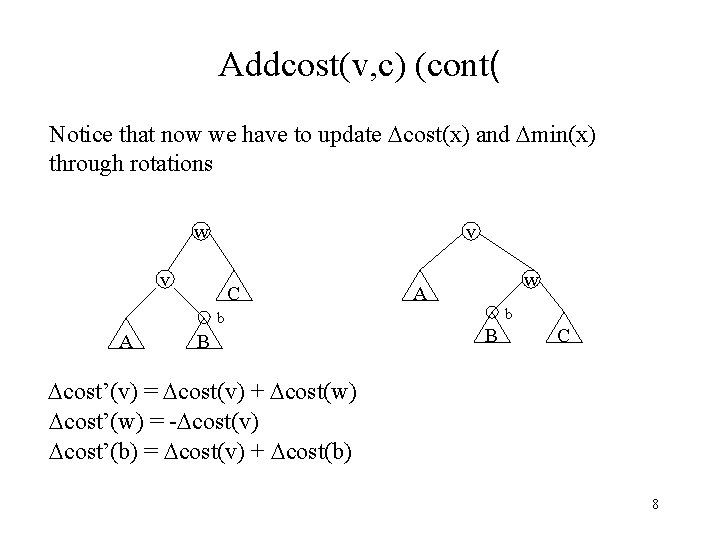Addcost(v, c) (cont( Notice that now we have to update cost(x) and min(x) through rotations w v v C b A B w A b B C cost’(v) = cost(v) + cost(w) cost’(w) = - cost(v) cost’(b) = cost(v) + cost(b) 8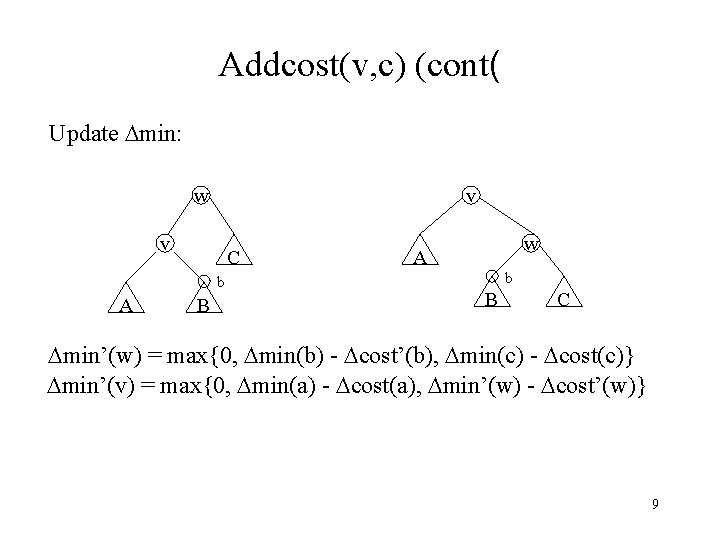Addcost(v, c) (cont( Update min: w v v C b A B w A b B C min’(w) = max{0, min(b) - cost’(b), min(c) - cost(c)} min’(v) = max{0, min(a) - cost(a), min’(w) - cost’(w)} 9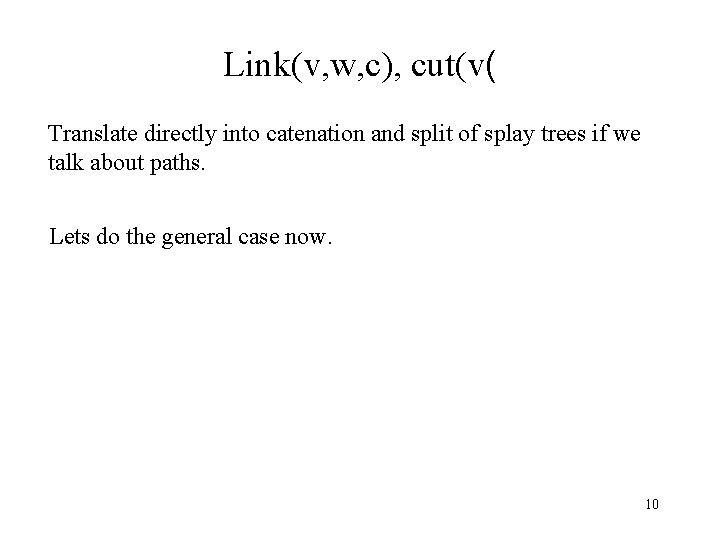Link(v, w, c), cut(v( Translate directly into catenation and split of splay trees if we talk about paths. Lets do the general case now. 10The virtual tree • We represent each tree T by a virtual tree V. The virtual tree is a binary tree with middle children. left middle right Think of V as partitioned into solid subtrees connected by dashed edges What is the relation between V and T ? 11Actual tree a b c d f e g i h j l k m r n o p q s t u v w 12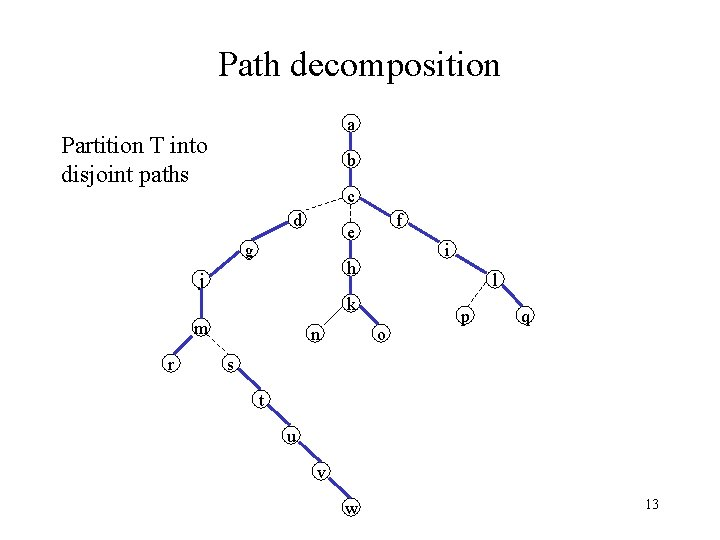Path decomposition a Partition T into disjoint paths b c d f e g i h j l k m r n o p q s t u v w 13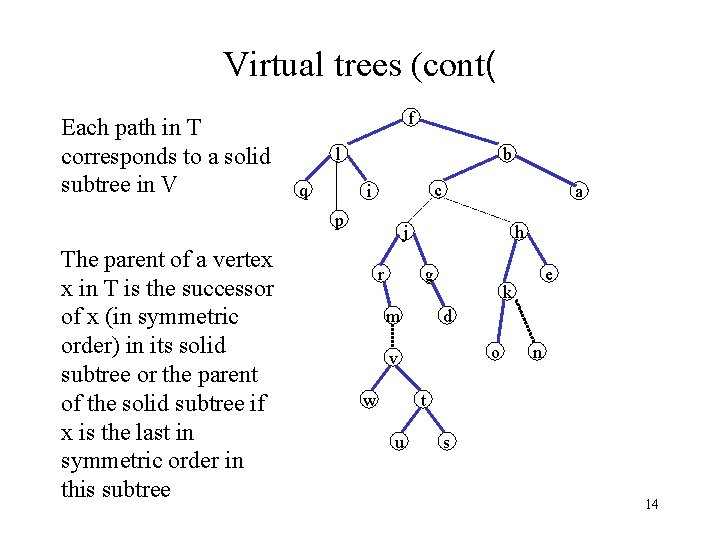Virtual trees (cont( Each path in T corresponds to a solid subtree in V f l q b c i p The parent of a vertex x in T is the successor of x (in symmetric order) in its solid subtree or the parent of the solid subtree if x is the last in symmetric order in this subtree a j r h g m k d o v w e n t u s 14Virtual trees (cont( f a l b q c d f e g r n p o p a j r l k m c i i h j b q h g m k d o v s t w u e n t u s v w 15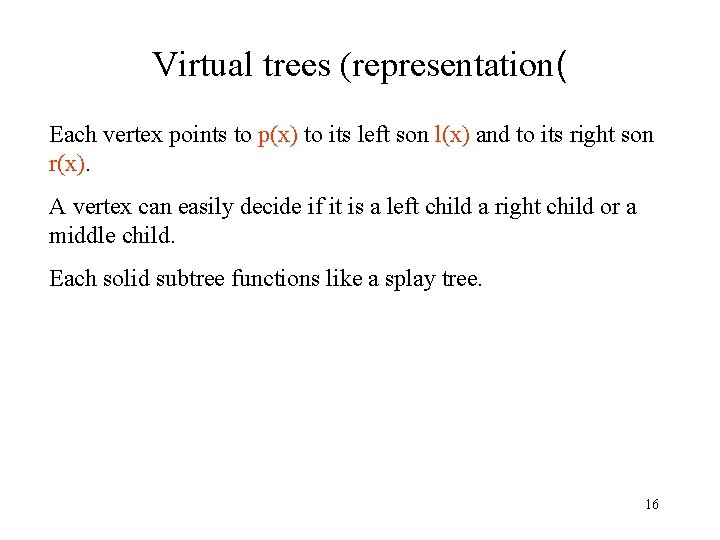Virtual trees (representation( Each vertex points to p(x) to its left son l(x) and to its right son r(x). A vertex can easily decide if it is a left child a right child or a middle child. Each solid subtree functions like a splay tree. 16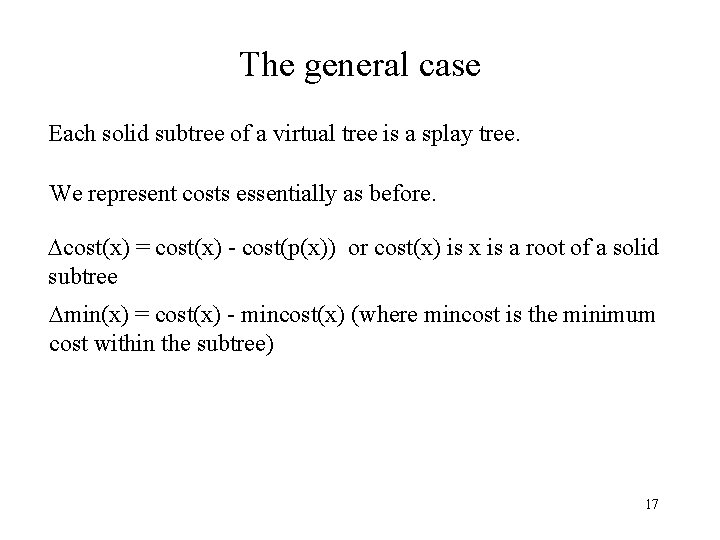The general case Each solid subtree of a virtual tree is a splay tree. We represent costs essentially as before. cost(x) = cost(x) - cost(p(x)) or cost(x) is x is a root of a solid subtree min(x) = cost(x) - mincost(x) (where mincost is the minimum cost within the subtree) 17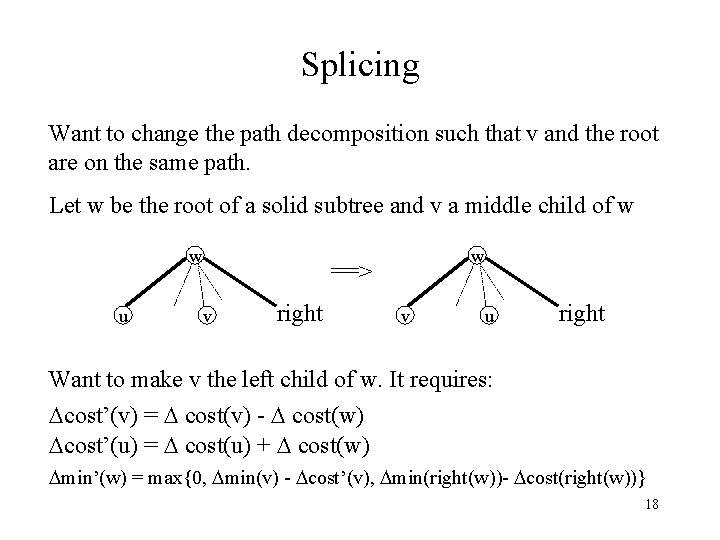Splicing Want to change the path decomposition such that v and the root are on the same path. Let w be the root of a solid subtree and v a middle child of w w u w ==> v right v u right Want to make v the left child of w. It requires: cost’(v) = cost(v) - cost(w) cost’(u) = cost(u) + cost(w) min’(w) = max{0, min(v) - cost’(v), min(right(w))- cost(right(w))} 18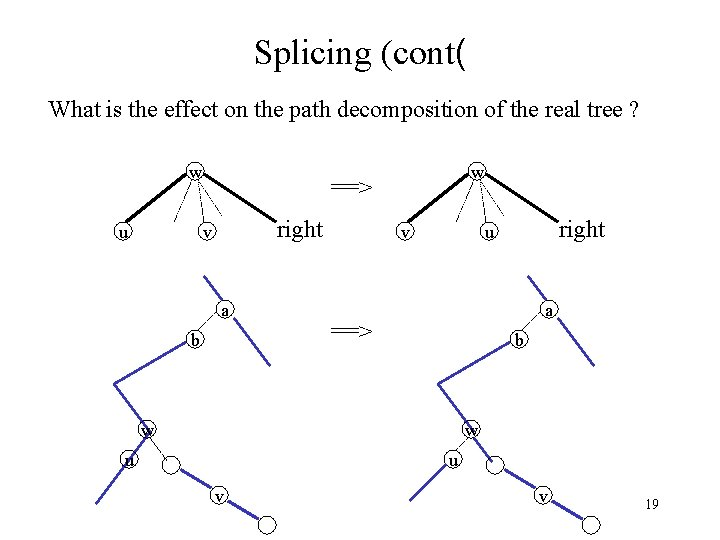Splicing (cont( What is the effect on the path decomposition of the real tree ? w u w ==> right v a b v right u a ==> b w w u u v v 19Splaying the virtual tree Let x be the vertex in which we splay. We do 3 passes: 1) Walk from x to the root and splay within each solid subtree After the first pass the path from x to the root consists entirely of dashed edges v w x 2) Walk from x to the root and splice at each proper ancestor of x. Now x and the root are in the same solid subtree 3) Splay at x Now x is the root of the entire virtual tree. 20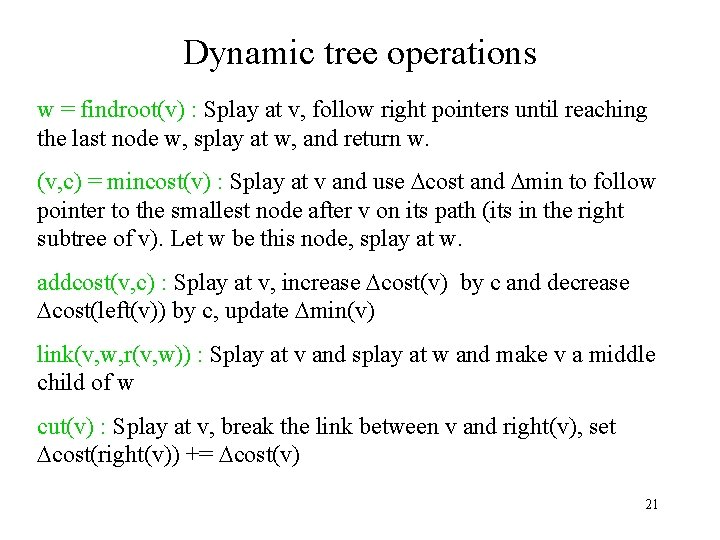Dynamic tree operations w = findroot(v) : Splay at v, follow right pointers until reaching the last node w, splay at w, and return w. (v, c) = mincost(v) : Splay at v and use cost and min to follow pointer to the smallest node after v on its path (its in the right subtree of v). Let w be this node, splay at w. addcost(v, c) : Splay at v, increase cost(v) by c and decrease cost(left(v)) by c, update min(v) link(v, w, r(v, w)) : Splay at v and splay at w and make v a middle child of w cut(v) : Splay at v, break the link between v and right(v), set cost(right(v)) += cost(v) 21Dynamic tree (analysis( It suffices to analyze the amortized time of splay. An extension of the access lemma. • Assign weight 1 to each node. The size of a node is the total number of descendants it has in the virtual tree. Rank is the log of the size. Potential is c times the sum of the ranks for some constant c. (So we can charge more than 1 for each rotation) xk x 1 x 22Dynamic tree (analysis( pass 1 takes 3 clogn + k pass 2 takes k k=#dashed edges on the path pass 3 takes 3 clogn + 1 – (c-1)(k-1) 23Proof of the access lemma (cont( y (3) zig ==> x A x C B y A B C amortized time(zig) = 1 + r’(x) + r’(y) - r(x) - r(y) 1 + r’(x) - r(x) 1 + 3(r’(x) - r(x)) 24Proof of the access lemma (cont( ==> y x A x z (1) zig - zig D y A C z B B C D amortized time(zig-zig) = 2 + r’(x) + r’(y) + r’(z) - r(x) - r(y) - r(z) = 2 + r’(y) + r’(z) - r(x) - r(y) 2 + r’(x) + r’(z) - 2 r(x) 2 r’(x) - r’(z) + r’(x) + r’(z) - 2 r(x) = 3(r’(x) - r(x)) 25Proof of the access lemma (cont( z (2) zig - zag x ==> y x A B y D A z B C D C Similar. (do at home) 26